# Model Evaluation API Examples¶

This demonstrates how to use the evaluate_model API.

Refer to the Model Evaluation guide for more details.

NOTES:

## Install MLTK Python Package¶

```# Install the MLTK Python package (if necessary)
```

## Import Python Packages¶

```# Import the necessary MLTK APIs
from mltk.core import evaluate_model
```

## Example 1: Evaluate Classification .h5 Model¶

The following example evaluates the image_example1 model using the `.h5` (i.e. non-quantized, float32 weights) model file.
This is a “classification” model, i.e. given an input, the model predicts to which “class” the input belongs.

The model archive is updated with the evaluation results.
Additionally, the `show` option displays interactive diagrams.

```# Evaluate the .h5 image_example1 model and display the results
evaluation_results = evaluate_model('image_example1', show=True)
print(f'{evaluation_results}')
```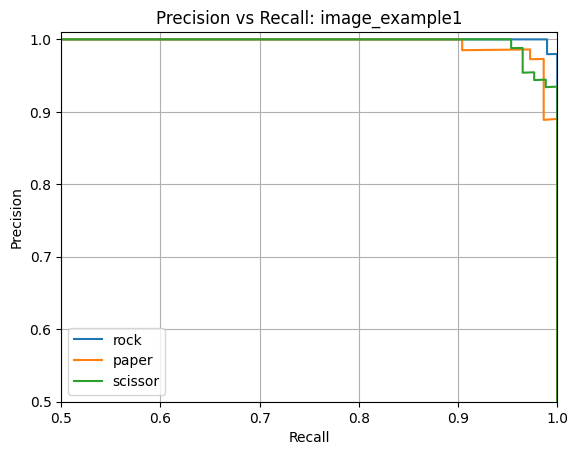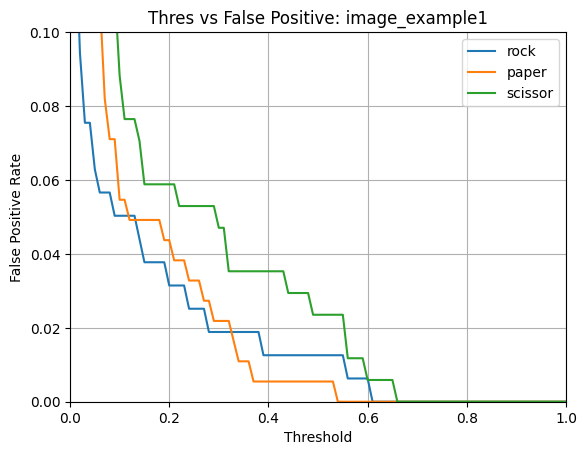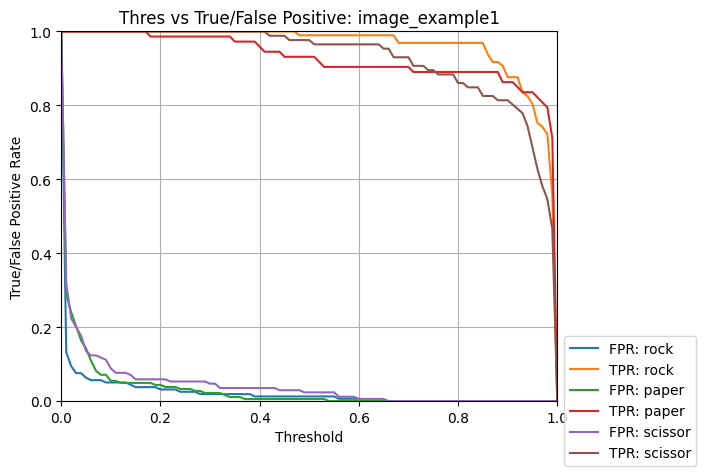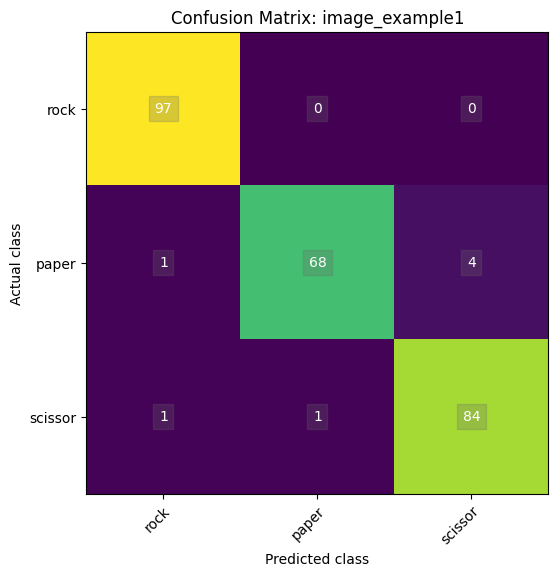```Name: image_example1
Model Type: classification
Overall accuracy: 97.266%
Class accuracies:
- rock = 100.000%
- scissor = 97.674%
- paper = 93.151%
Average ROC AUC: 99.919%
Class ROC AUC:
- rock = 99.987%
- scissor = 99.891%
- paper = 99.880%
```

## Example 2: Evaluate Classification .tflite Model¶

The following example evaluates the image_example1 model using the `.tflite` (i.e. quantized, int8 weights) model file.
This is a “classification” model, i.e. given an input, the model predicts to which “class” the input belongs.

The model archive is updated with the evaluation results.
Additionally, the `show` option displays interactive diagrams.

```# Evaluate the .tflite image_example1 model and display the results
evaluation_results = evaluate_model('image_example1', tflite=True, show=True)
print(f'{evaluation_results}')
```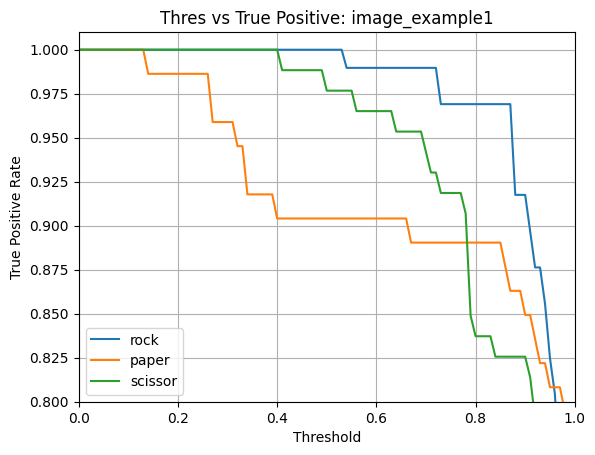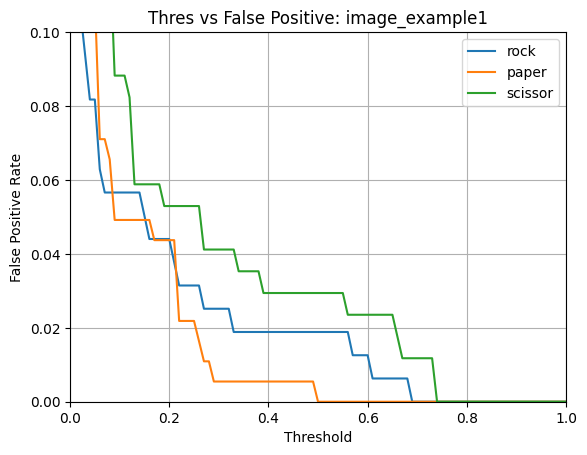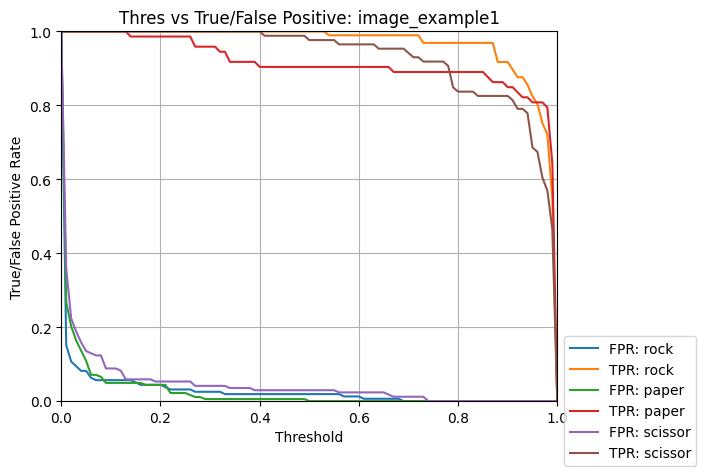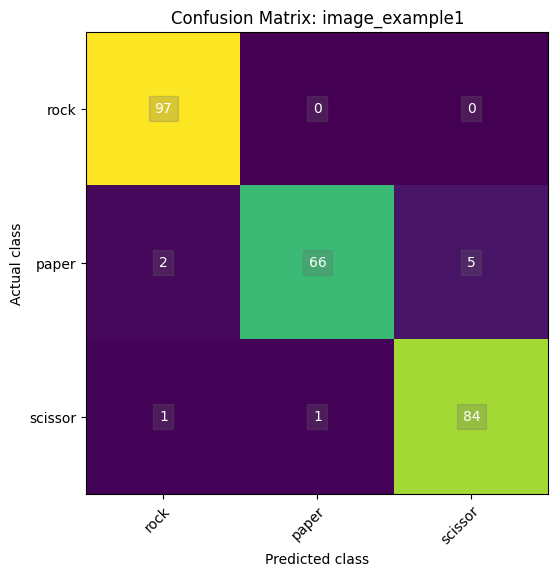```Name: image_example1
Model Type: classification
Overall accuracy: 96.484%
Class accuracies:
- rock = 100.000%
- scissor = 97.674%
- paper = 90.411%
Average ROC AUC: 99.893%
Class ROC AUC:
- rock = 99.981%
- paper = 99.865%
- scissor = 99.832%
```

## Example 3: Evaluate Auto-Encoder .h5 Model¶

The following example evaluates the anomaly_detection model using the `.h5` (i.e. non-quantized, float32 weights) model file.
This is an “auto-encoder” model, i.e. given an input, the model attempts to reconstruct the same input. The worse the reconstruction, the further the input is from what the model was trained against, this indicates that an anomaly may be detected.

The model archive is updated with the evaluation results.
Additionally, the `show` option displays interactive diagrams and the `dump` option dumps a comparison image between the input and reconstructed image.

```# Evaluate the .h5 anomaly_detection model and display the results
evaluation_results = evaluate_model('anomaly_detection', show=True, dump=True)
print(f'{evaluation_results}')
```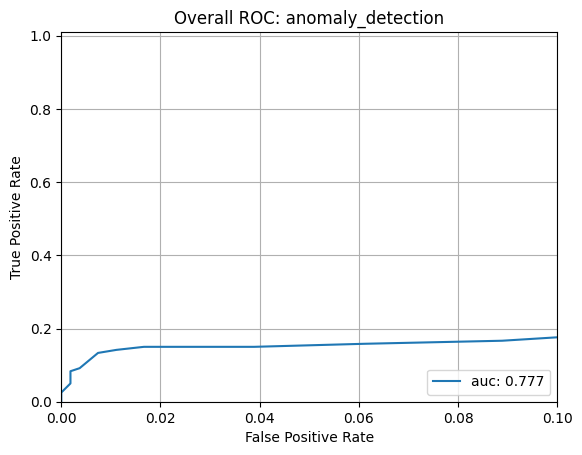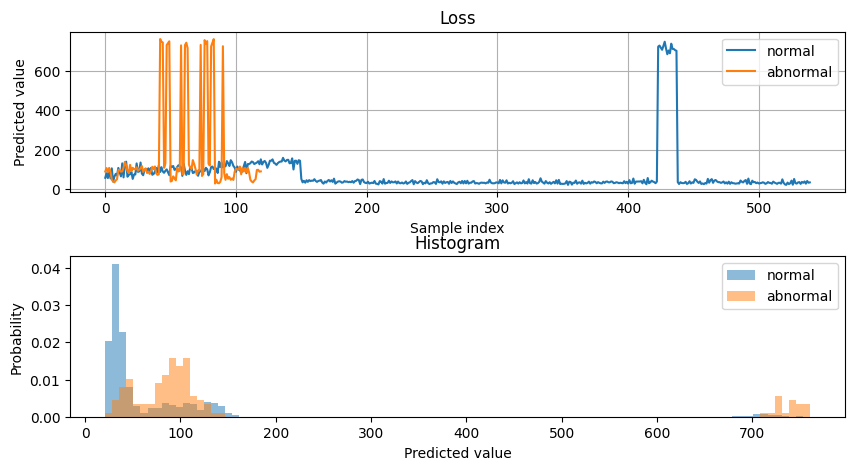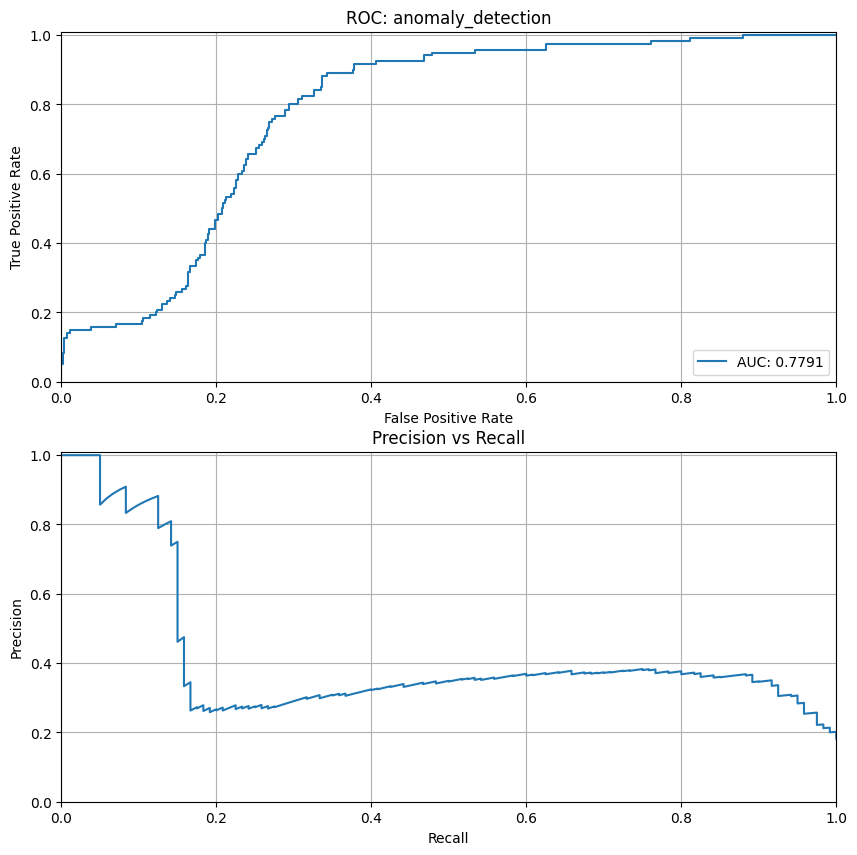```Name: anomaly_detection
Model Type: auto_encoder
Overall accuracy: 83.636%
Precision/recall accuracy: 62.308%
Overall ROC AUC: 77.726%
```